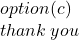20 POINTS which of the following describes newton’s third law of motion? A) Force is equal to mass of an object multiplied by it

Question

20 POINTS
which of the following describes newton’s third law of motion?
A) Force is equal to mass of an object multiplied by its acceleration (F=m*a)
B) An object at rest stays at rest unless acted upon by an outside force
C) For every action, there is an equal and opposite reaction

in progress 0
6 months 2021-07-28T14:55:19+00:00 2 Answers 11 views 0

C) For every action, there is an equal and opposite reaction

Explanation:

Newton’s Third Law

For every force, there is an equal and opposite reaction time. Explains why forces act in pairs.

Brainliest?